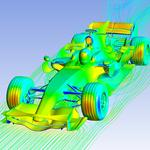# What is the equation or formula to calculate Mass Flux[kg/m²s] of a species in Fluent before running calculation?

Hello! In Fluent, I am curious about how to calculate mass flux of a species [kg/m²s] from the mass fraction of that species which has density=0.25kg/m³, that species was applied to a Wall BC (Horizontal line), there are no Normal velocities (Y-velocity) on that wall, but there are axial velocities (X-velocity) on that Wall boundary. That species has a Molecular weight=6kg/kmol. Note that there is only one species on that Wall boundary. In fluent, to set up that wall boundary, there is also the species tap to let us to fill out the mass fraction, so I put mass fraction=1, because there is only one species on that boundary. Therefore, how can I calculate the mass flux of that species [kg/m²s] from the detailed information that I provide? Or if you want more information, I can provide you further. It is the equation for calculating mass flux before running calculation in fluent, because after running calculation, I will get the results and I will calculate mass flux from those results by the equation provided by fluent: Ji=((density*Diffusion coefficient of a species)+(density*Effective diffusion coefficient of turbulent flow))*mass fraction gradient, then take this results to compare with the flux before running calculation. In this problem, I just want to know the equation or formulas to calculate that mass flux of a species before getting the results from fluent. Thank you! I will appreciate your help.